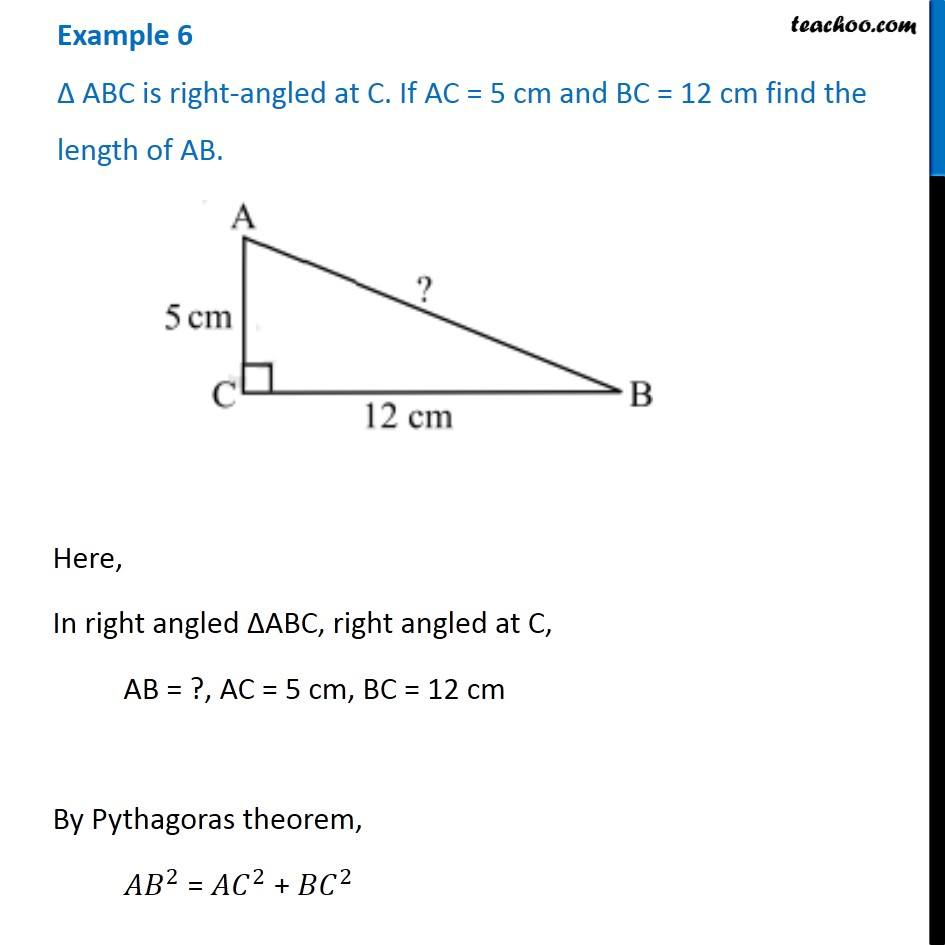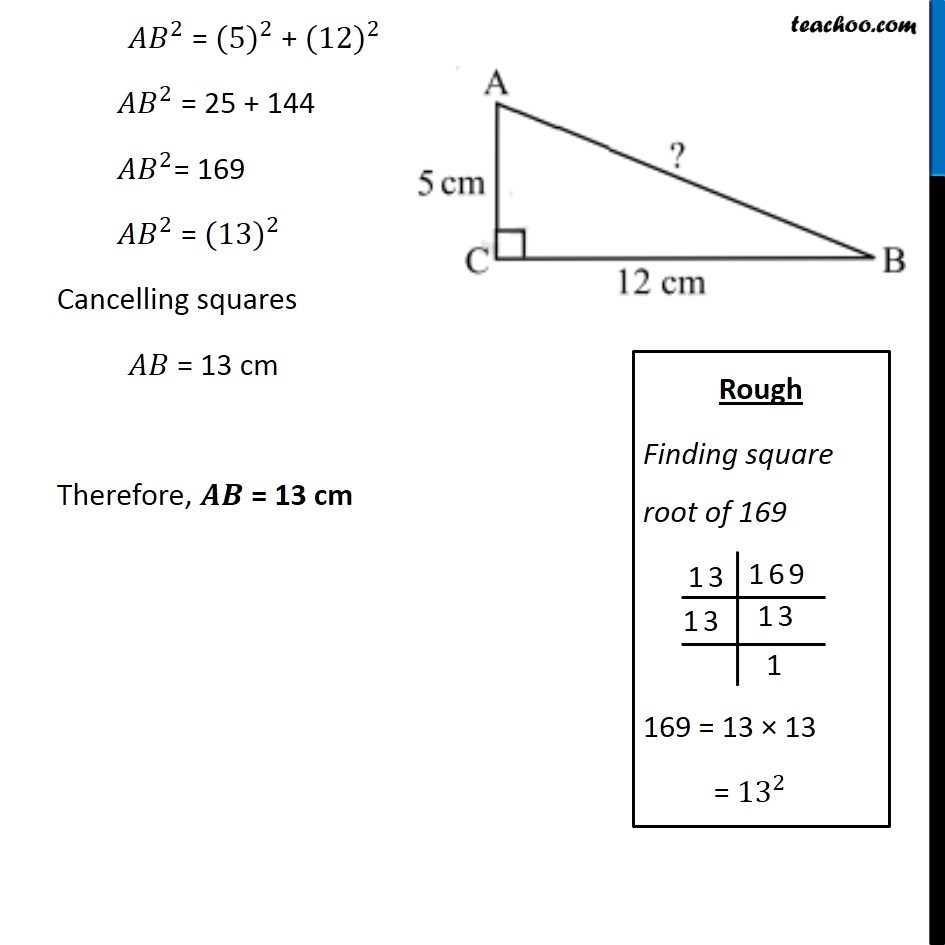Subscribe to our Youtube Channel - https://you.tube/teachoo

1. Chapter 6 Class 7 Triangle and its Properties
2. Concept wise
3. Pythagoras Theorem

Transcript

Example 6 ∆ ABC is right-angled at C. If AC = 5 cm and BC = 12 cm find the length of AB. Here, In right angled ∆ABC, right angled at C, AB = ?, AC = 5 cm, BC = 12 cm By Pythagoras theorem, 〖𝐴𝐵〗^2 = 〖𝐴𝐶〗^2 + 〖𝐵𝐶〗^2 〖𝐴𝐵〗^2 = 〖(5)〗^2 + 〖(12)〗^2 〖𝐴𝐵〗^2 = 25 + 144 〖𝐴𝐵〗^2= 169 〖𝐴𝐵〗^2 = 〖(13)〗^2 Cancelling squares 𝐴𝐵 = 13 cm Therefore, 𝑨𝑩 = 13 cm

Pythagoras Theorem